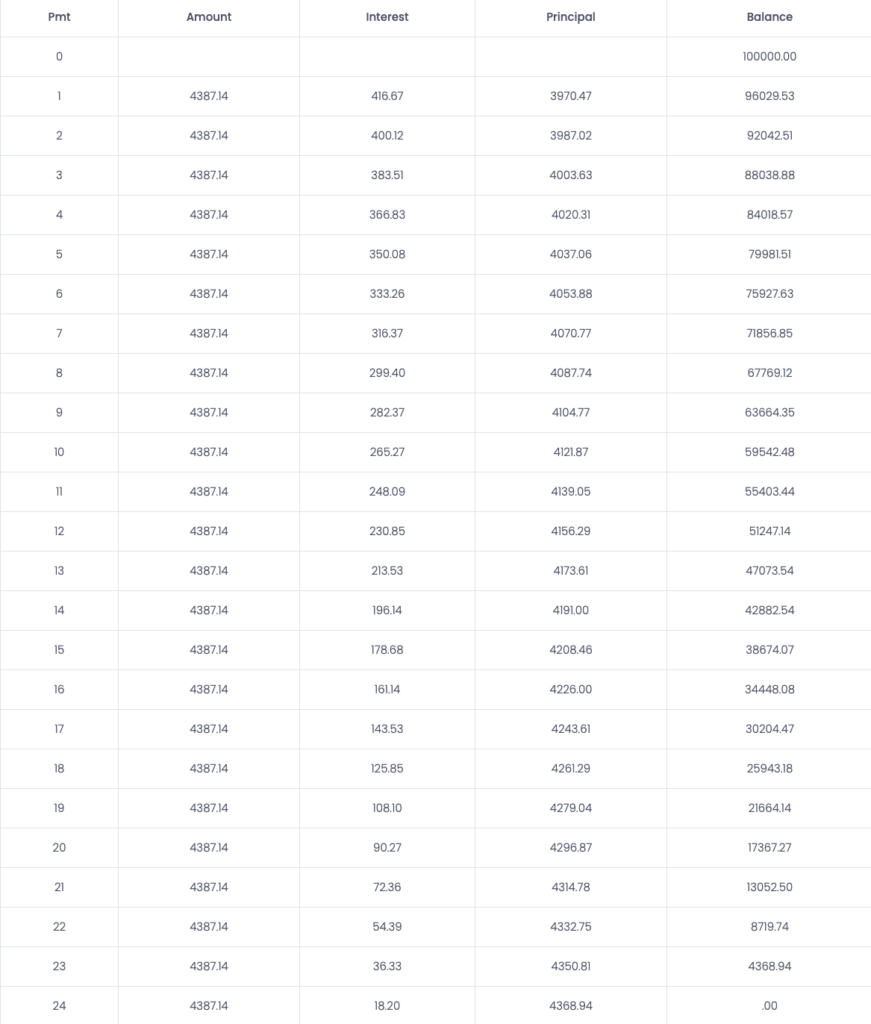# Description of Loan Amortization Calculator

Amortization is the gradual decrease of a debt over a set period of time. It is a process of spreading out a loan into fixed periodic payments. Each monthly payment will be fixed to a certain amount of the loan. However, a portion of the payment will cater to the requirements of loan costs, which the lender gets to pay for acquiring the loan and reducing the principal loan amount.

## How to Calculate Loan Amortization

While calculating the amortization of the loan, the payment is based on the amount of the loan, the total number of installments, and the annual interest rate charged. These three elements will help you evaluate your monthly payments and the interest fee.

Payment Formula: PMT=iPV/(1-(1+i)-n)

Here:

• PMT= total payment each period
• i= periodic interest rate
• PV= present value of the loan
• n= number of loan installments

Using the payment formula, we can evaluate the present value of each loan installment paid to the lender. The payment calculated will be the total payment each month for the duration of the loan. Loan payments consist of two parts: principal and interest.

Interest Payment Formula: P*i

Here:
P= Remaining Principal

i= interest rate

## Amortization Schedule Example

Let’s take an example to calculate the Loan Amortization. For instance, we take out a loan of 2-year, \$100,000 at 5% annually, with monthly payments.The first step here is to calculate the monthly payment towards the loan. We will use the above formula to insert the present value of the loan, interest rate and the total number of installments.

PMT= ((0.05/12)*100000)/(1-(1+0.05/12)^-24)

PMT= 4387

For each month, the total payment will be 4387. Next, we need to calculate the interest paid towards the loan each month.

Interest= 100,000 * 0.05/12

Interest= 416.67

It represents the payment paid towards the interest is \$416.67 in this first month. However, this value will vary each month according to the balance of the loan.

The next step is to calculate the portion paid towards the principal. This value will be determined using the formula below:

Principal= 4387.14 – 416.67

Principal= 3970.47

Lastly, the balance figure evaluates the balance of the loan after a period’s payment is the previous balance of the loan minus the portion of the payment made towards the principal. For the first month, the calculation will be:

Balance= 100,000- 3970.47

Balance= 96029.53

Now that we have all our values calculated, the schedule of payments will look like this:

##Common Types of Amortization Loans:

1. Personal Loans: These loans are accessible to get from banks, credit unions or online lenders. Borrowers are given out these amortized loans on mostly three-year periods, fixed interest rates and a fixed number of installments.
2. Auto Loans: Auto loans stretch to a period of less than a five-year period with a fixed number of installments over the period. Borrowers may avail for a longer period loan; however, extending the loan over a longer period may lower the payment, but the person would end up paying more in interest over the time. It may exceed the car’s resale value at the end of the period.
3. Home Loans: Home loans are based on fixed-rate mortgages of an over 15-year period. In this case, people often refinance the loan or sell the home instead of keeping the loan for a longer period of time.